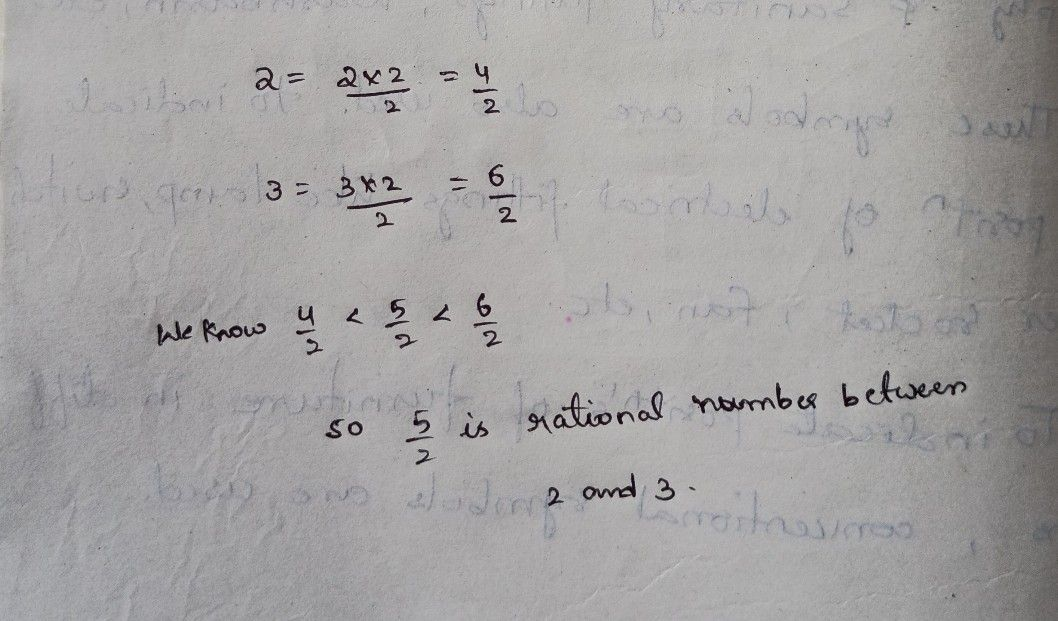Symbol
Problem$2.$ Find a rational number between $2$ and $3$ $-1$ $3.$ Find a rational number between $\bar{2}$ and $\dfrac {1} {2}$
7th-9th grade
Other
Search count: 112
SolutionQanda teacher - Sreejaplease evaluate it